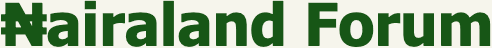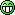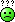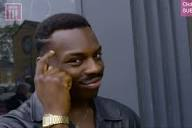#Welcome, Guest: Join Nairaland / LOGIN! / Trending / Recent / New
Stats: 2,619,955 members, 6,104,840 topics. Date: Monday, 18 January 2021 at 07:17 AM

## Why Is Any Number Multiplied By Zero Equals To Zero? - Education - Nairaland

 Why Is Any Number Multiplied By Zero Equals To Zero? by BobFischer(m): 10:15am On Jan 13 Why is any any number multiply by zero is equal to zero? 10 x 0 = 0100 x 0 = 01000 x 0 = 0If i have 100 oranges on a table and i multiply it by zero. Why do i suddenly have zero?100 oranges X 0 = 01000 pears X 0 = 0front page pleasemathematicians in the house please gather. 33 Likes 2 Shares Re: Why Is Any Number Multiplied By Zero Equals To Zero? by eddynaira125: 10:24am On Jan 13 This are mathematical laws that u should obey,U can't ask God y thou shall not kill2*3= lll lll. Three in two places0*3 =. Means 3 in zero placesThat's is y we arrive there as our final ans 521 Likes 27 Shares Re: Why Is Any Number Multiplied By Zero Equals To Zero? by sinkhole: 10:29am On Jan 13 BobFischer:Why is any any number multiply by zero is equal to zero? 10 x 0 = 0100 x 0 = 01000 x 0 = 0If i have 100 oranges on a table and i multiply it by zero. Why do i suddenly have zero?100 oranges X 0 = 01000 pears X 0 = 0front page pleasemathematicians in the house please gather.add no orange (0) ten times (10x), that is 10x0, what will you get?ORadd ten oranges at no time, what will you get?(Note that multiplication is simply repeated addition) 185 Likes 10 Shares Re: Why Is Any Number Multiplied By Zero Equals To Zero? by BobFischer(m): 10:56am On Jan 13 eddynaira125:This are mathematical laws that u should obey,U can't ask God y thou shall not kill2*3= lll lll. Three in two places0*3 =. Means 3 in zero placesThat's is y we arrive there as our final ansmind you 0 * 3 and 3 * 0 are two different statement. 0 (nothing) * 3 (something) = 0 (nothing)3 (something) * 0 (nothing) = 0 (nothing).why isnt it 3 * 0 = 30 * 3 = 0 107 Likes 5 Shares Re: Why Is Any Number Multiplied By Zero Equals To Zero? by Worldliness(f): 11:33am On Jan 13 BobFischer:Why is any any number multiply by zero is equal to zero? 10 x 0 = 0100 x 0 = 01000 x 0 = 0If i have 100 oranges on a table and i multiply it by zero. Why do i suddenly have zero?100 oranges X 0 = 01000 pears X 0 = 0front page pleasemathematicians in the house please gather.Zero practically means nothing.So nothing 1 time, nothing 2 times and nothing 1000 times 0r 1million times is still equal to nothing.Eg) if i dont have 1 naira, if you ask me for 1 naira for 1 time or even 100 times, i still dont have any. that is it. 375 Likes 21 Shares Re: Why Is Any Number Multiplied By Zero Equals To Zero? by paulolee(m): 11:40am On Jan 13 asked my maths for economics lecturer this question when he was taking us matrix during year1 and the whole class burst into laughter amd the prof sent me.out of his class..I gat go beg the man with a bottle of wine and 2k recharge card, the man still deduct 5 marks from me for taking his class for a ride just because of this same logical question..dnt jus know why our uni lecturers do feel like demi gods.. 193 Likes 8 Shares Re: Why Is Any Number Multiplied By Zero Equals To Zero? by proxitaly: 12:03pm On Jan 13 Buhari (something) x Buhari brain (empty or nothing) = nothingThat is why even 1000 tenures of Buhari will leave Naija empty or even worse off. You grab? 276 Likes 28 Shares Re: Why Is Any Number Multiplied By Zero Equals To Zero? by Fortune109: 1:07pm On Jan 13 It's just simple English or any other languageThey said...If you don't have 100, what do you have??If you don't have 1000, what do you have??Answer those questions and get your answer! 14 Likes 1 Share Re: Why Is Any Number Multiplied By Zero Equals To Zero? by Tohsynetita1: 1:50pm On Jan 13 BobFischer:Why is any any number multiply by zero is equal to zero? 10 x 0 = 0100 x 0 = 01000 x 0 = 0If i have 100 oranges on a table and i multiply it by zero. Why do i suddenly have zero?100 oranges X 0 = 01000 pears X 0 = 0front page pleasemathematicians in the house please gather. Probably, you don't understand the meaning of multiplication, God says be fruitful and multiply. For multiplication to occur, it must be 2 entity that are not empty, Procreation is as a result of union of two people. Man with Nothing can not bring a child. The zero, in the expression will always affect the answer and make it to be nothing. 63 Likes 6 Shares Re: Why Is Any Number Multiplied By Zero Equals To Zero? by dawnomike(m): 2:02pm On Jan 13 eddynaira125:This are mathematical laws that u should obey,U can't ask God y thou shall not kill2*3= lll lll. Three in two places0*3 =. Means 3 in zero placesThat's is y we arrive there as our final ansThe branch of mathematics that proves this is called 'Real Analysis'. You proof by contradiction. To proof what you asked above in mathematics will take like 2 pages of paper. 34 Likes 2 Shares Re: Why Is Any Number Multiplied By Zero Equals To Zero? by pat077: 2:04pm On Jan 13 U can't put something on nothing and expect it to stand. 18 Likes 1 Share Re: Why Is Any Number Multiplied By Zero Equals To Zero? by BobFischer(m): 2:20pm On Jan 13 Worldliness:Eg) if i dont have 1 naira, if you ask me for 1 naira for 1 time or even 100 times, i still dont have any. that is it.This translate to zero 0 (if i dont have 1 naira) * 1 or * 100 = 0 (i still dont have any)NOW THIS(You have) 100 * 0 (nothing) = 0 (nothing)translate to you have 100 and i ask zero time(simply i dont ask) and then you loose your 100so how do you explain this?losing you 100 when you do nothing 11 Likes Re: Why Is Any Number Multiplied By Zero Equals To Zero? by VinceSpence(m): 2:31pm On Jan 13 3 * 0 and 0 * 3 are the same, sir. Multiplication is commutative. 37 Likes 3 Shares Re: Why Is Any Number Multiplied By Zero Equals To Zero? by nnamdiosu(m): 2:32pm On Jan 13 proxitaly:Buhari (something) x Buhari brain (empty or nothing) = nothingThat is why even 1000 tenures of Buhari will leave Naija empty or even worse off. You grab?stop na 22 Likes 2 Shares Re: Why Is Any Number Multiplied By Zero Equals To Zero? by BobFischer(m): 2:38pm On Jan 13 pat077:U can't put something on nothing and expect it to stand. yet the sun stand on nothingpat077:U can't put something on nothing and expect it to stand. yet the sun stand on nothing 10 Likes Re: Why Is Any Number Multiplied By Zero Equals To Zero? by BobFischer(m): 2:41pm On Jan 13 dawnomike:The branch of mathematics that proves this is called 'Real Analysis'. You proof by contradiction. To proof what you asked above in mathematics will take like 2 pages of paper.PLEASE HELP US WITH IT 2 Likes Re: Why Is Any Number Multiplied By Zero Equals To Zero? by BobFischer(m): 2:43pm On Jan 13 paulolee:asked my economics lecturer the same during year1 n the whole class burst into laughter amd the prof sent me.out of his classhahahahaha. What happens when you start questioning the system. Sorry for being treated unfairly 4 Likes Re: Why Is Any Number Multiplied By Zero Equals To Zero? by smartty68(m): 2:58pm On Jan 13 0 * 10 oranges at hand = 10 oranges... Reasons being that the oranges won't mysteriously disappear from my hand...Imagine multiplying the 6 luggage bags by 0 and yet the load from the luggage still on you... 27 Likes 2 SharesRe: Why Is Any Number Multiplied By Zero Equals To Zero? by Cheato64(m): 3:20pm On Jan 13 BobFischer:Why is any any number multiply by zero is equal to zero? 10 x 0 = 0100 x 0 = 01000 x 0 = 0If i have 100 oranges on a table and i multiply it by zero. Why do i suddenly have zero?100 oranges X 0 = 01000 pears X 0 = 0front page pleasemathematicians in the house please gather. if you and 2 of your friends are giving 0 mangoes to share, how many will each get? 14 Likes 1 Share Re: Why Is Any Number Multiplied By Zero Equals To Zero? by Spy360(m): 4:03pm On Jan 13 Worldliness:Zero practically means nothing.So nothing 1 time, nothing 2 times and nothing 1000 times 0r 1million times is still equal to nothing.Eg) if i dont have 1 naira, if you ask me for 1 naira for 1 time or even 100 times, i still dont have any. that is it. 6 Likes 1 ShareRe: Why Is Any Number Multiplied By Zero Equals To Zero? by Mrshape: 4:28pm On Jan 13 Because multiplication is addition.0x2 means add 0 two times 0+0=00×5 means add zero five times 0+0+0+0+0=0 38 Likes 5 Shares Re: Why Is Any Number Multiplied By Zero Equals To Zero? by BobFischer(m): 4:32pm On Jan 13 VinceSpence:3 * 0 and 0 * 3 are the same, sir. Multiplication is commutative.1 + 2 = 3.2 + 1 = 3. The two statement above is not the same though the result is the same 3 Likes Re: Why Is Any Number Multiplied By Zero Equals To Zero? by maynia: 5:04pm On Jan 13 proxitaly:Buhari (something) x Buhari brain (empty or nothing) = nothingThat is why even 1000 tenures of Buhari will leave Naija empty or even worse off. You grab?4 Likes 2 Shares Re: Why Is Any Number Multiplied By Zero Equals To Zero? by BigDawsNet: 5:42pm On Jan 13 Zero to the power of zero, denoted by 00, is a mathematical expressionZero no dey take anybody play 2 Likes Re: Why Is Any Number Multiplied By Zero Equals To Zero? by everglaze01: 5:49pm On Jan 13 If somebody send u an alert of 0 naira 100 times. How much do u now have? 31 Likes 5 Shares Re: Why Is Any Number Multiplied By Zero Equals To Zero? by CalliDora1(f): 6:23pm On Jan 13 My own reasoning is thisWe all know 0 is nothing. Now if you put nothing in ten places, youll get nothing in those ten places. Whic equals to =0/ nothing. So here 10 is represented by the places a number occurs.Hope I've not ended up confusing you further. 8 Likes 1 Share Re: Why Is Any Number Multiplied By Zero Equals To Zero? by Stephenmoka4(m): 6:41pm On Jan 13 BobFischer:Why is any any number multiply by zero is equal to zero? 10 x 0 = 0100 x 0 = 01000 x 0 = 0If i have 100 oranges on a table and i multiply it by zero. Why do i suddenly have zero?100 oranges X 0 = 01000 pears X 0 = 0front page pleasemathematicians in the house please gather. How many times should zero appear?10 times of zero is zero, is like giving depositing 0 ten times, why is still an empty space. Hence, zero 1 Like Re: Why Is Any Number Multiplied By Zero Equals To Zero? by Deathissweet: 6:47pm On Jan 13 B Re: Why Is Any Number Multiplied By Zero Equals To Zero? by waynetee(m): 6:50pm On Jan 13 This explains everything, i dont know what elseeddynaira125:This are mathematical laws that u should obey,U can't ask God y thou shall not kill2*3= lll lll. Three in two places0*3 =. Means 3 in zero placesThat's is y we arrive there as our final ans 1 Like 1 Share Re: Why Is Any Number Multiplied By Zero Equals To Zero? by STINOS(m): 6:52pm On Jan 13 First the commonsense explanation:Say you are giving away free apples. An infinitely big apple tree has sprouted in your garden (it's hypothetical) and you have decided to give away apples to each person who comes by. You have decided that you'll give 1 apple to each person that comes by. If one person comes by, you have given away one apple. If two people come by, you have given away two apples. If seventy-five people come by you have given away seventy-five apples. This is multiplication by 1. If there are n people that come by, 1*n tells you how many apples you have given away.Say you are giving away 2 apples per person. If one person comes by, you have given away two apples. If two people come by you have given away four apples. If thirty people come by you have given away sixty apples. This is multiplication by 2. So if 'n' is how many people came by, 2*n tells you how many apples you have given away.Similarly, if you are giving away 100 apples per person, 100*n tells you how many apples you have given away in total where 'n' is how many people came by to collect their apples (everyone loves apples).If you are giving away 0 apples per person, then regardless of how many people come by, how many apples would you have given out by the end of the day? Logically? None, of course! Hence, 0*n = 0.This also works the other way around, because of the commutative law for multiplication. So lets say no people come by, i.e., n = 0. Regardless of how many apples you want to give away, the total number of apples you have given away will always be 0. Cause no one comes by to take the apples! So a*0 = 0 where a is how many apples you give away per person, which doesn't matter.Now for an explanation hinging slightly more on mathematical notation:Multiplication is basically addition, done over and over again. Multiplying a number n by 2 means adding n twice --> n + nMultiplying a number by 7 means adding it seven times --> n + n + n + n + n + n + nMultiplying a number a gazillion times means n + n + n ... (a gazillion n's)So what you multiply 'n' by tells you the number of n you have to add together. If you multiply n by 0, then there are no n's in the picture. Since you are not adding anything, you are left with nothing, aka 0. So:2*n = n + n (two n's on this side)7*n = n + n + n + n + n + n + n (seven n's on this side)0*n = (how many n's do you see on this side? None. 88 Likes 9 Shares Re: Why Is Any Number Multiplied By Zero Equals To Zero? by VictorUSA: 7:30pm On Jan 13 eddynaira125:This are mathematical laws that u should obey,U can't ask God y thou shall not kill2*3= lll lll. Three in two places0*3 =. Means 3 in zero placesThat's is y we arrive there as our final ansBrilliant answer Re: Why Is Any Number Multiplied By Zero Equals To Zero? by Stubborn82: 7:30pm On Jan 13 Am I the only person who noticed the op must be a genius A question like this is not just a question for fun, in fact it is a question born of deep provoking thought.Luke Williams called this kind a of question, a disruptive question that need a disruptive solution, this is how great ideas is born.When you try to turn upside down or turn back from the norm, you become genius. OP please be my friend, I need a great thinkers like you to share mind with. 26 Likes 4 Shares

(Go Up)

 Sections: politics (1) business autos (1) jobs (1) career education (1) romance computers phones travel sports fashion health religion celebs tv-movies music-radio literature webmasters programming techmarket Nairaland - Copyright © 2005 - 2021 Oluwaseun Osewa. All rights reserved. See How To Advertise. 117Disclaimer: Every Nairaland member is solely responsible for anything that he/she posts or uploads on Nairaland.